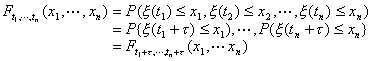§3  随机过程

[随机过程的定义]  对于每个tÎT(T 是某个固定的实数集),x (t)是个随机变量，就把这样的随机变量族{x (t)tÎT}称为随机过程。随机过程一次实验的结果是定义在T上的函数，称为随机过程的一次实现。当参数t的变化范围T是个整数集合，则称

x (t), t=0,±1,±2,L

T只包含一个或有限个元素，{x (t)tÎT}就是概率论中研究过的随机变量或随机矢量。

[随机过程的有穷维分布函数族]  {x (t)tÎT}是随机过程，对任意的正整数n及任意的t1, t2, LtnÎT,随机变量x (t1) ,x (t2) ,Lx (tn)的联合分布函数为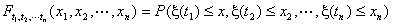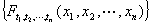为随机过程的有穷维分布函数族。它不仅刻划了对应于每一个t的随机变量x(t)的统计规律性，而且也刻划了各个随机变量x(t)之间的关系，从而完整地描述了随机过程的统计规律性。

[随机过程的统计参数]  {x (t)tÎT}是个复值随机过程(指它的实部和虚部都是实的随机过程)。主要的统计参数有：

1° 均值函数  对每个tÎT,随机变量x(t)的数学期望（均值）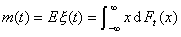2° 协方差函数与方差函数  对任意的s, tÎT,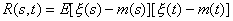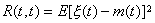3° 高阶矩  若对任意的正整数n，非负整数m1 , m2 ,L , mn , m= m1+m2+L+mn及任意实数t1,t2,L,tn随机变量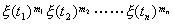的数学期望存在，则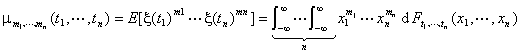[随机过程的均方连续性]  {x (t)tÎT}是一随机过程，t0ÎT,如果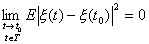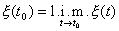1° 随机过程{x (t)tÎT}T上均方连续；

2° 随机过程{x (t)tÎT}的协方差函数R(s,t)(s,tÎT)关于s,t是连续的；

3° 随机过程{x (t)tÎT}协方差函数R(s,t)(s,tÎT)在对角线s=t上关于s,t是连续的。

[独立随机过程]  若对任意的正整数n和任意的t1,t2,LtnÎT,随机变量x (t1),x (t2),L,x (tn)是相互独立的，即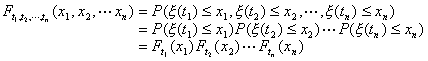[正态过程]  若对任意的正整数n和任意的t1,t2,L,tnÎT,随机变量x (t1),x (t2),L,x (tn)的联合分布总是正态的，即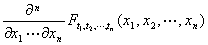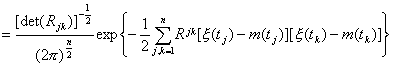[马尔科夫过程]  若对任意的n=1,2,L和任意的t0,t1,L,tnÎT(其中t0<t1<L<tn)以及任意的实数x, y等式

P{x (tn)y|x (tn-1)=x,x (tn-2)=xn-2,L,x (t0)=x0}=P{x (tn)y|x (tn-1)=x}

[时齐马尔科夫过程]  {x (t)tÎT}是马尔科夫过程，若对任意的t1ÎT,t2ÎT

(t1<t2),条件分布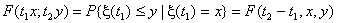[具有独立增量的随机过程]  若对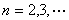及任意一组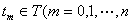，其中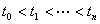），随机变量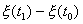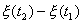¼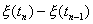是相互独立的，则称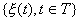是个具有独立增量的随机过程。

[具有平稳增量的随机过程]  若对任意的t1,t2ÎT和任意h(t1+h,t2+hÎT)，随机变量

x (t2+h)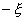(t1+h)x(t2)(t1)

[泊松过程]  {x (t)0t<}是具有平稳独立增量，取非负整数值的随机过程。如果对于任意t (0t<),关系式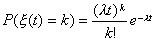(k=0,1,2,L)

[维纳过程]  若随机过程{x (t)0t<}满足P(x (0)=0)=1,具有平稳独立增量，并且随机变量x (t)的分布密度函数是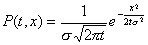[平稳过程]  若对于n=1,2,L,任意tmÎT(m=1,2,L,n)及任意的τ(tm+τÎT,m=1,2,L,n)，等式